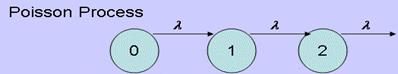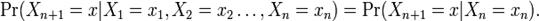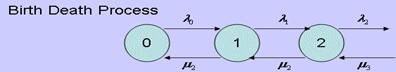Published on Mar 28, 2020

### Abstract

Queuing Theory is a collection of mathematical models of various queuing systems. It is used extensively to analyze production and service processes exhibiting random variability in market demand (arrival times) and service times.

In queuing theory , a queuing model is used to approximate a real queuing situation or system, so the queuing behavior can be analyzed mathematically. Queuing models allow a number of useful steady state performance measures to be determined, including:

The Average number in the queue, or the system, the average time spent in the queue, or the system, the statistical distribution of those numbers or times, the probability the queue is full, or empty, and the probability of finding the system in a particular state.

These performance measures are important as issues or problems caused by queuing situations are related to customer dissatisfaction with service or may be the root cause of economic losses in a business. Analysis of the relevant queuing models allows the cause of queuing issues to be identified and the impact of proposed changes to be assessed.

### Markovian queueing model :-

If the arrival process is Poisson and the service time distributions are exponential for a queuing model then it is said to be a Markovian queuing model .A Markov chain is a sequence of random variables X 1 , X 2 , X 3 , ... with the Markov property , namely that, given the present state, the future and past states are independent. Formally,### Birth-death process :

The birth-death process is a special case of continuous-time Markov process where the states represent the current size of a population and where the transitions are limited to births and deaths. Birth-death processes have many applications in demography, queuing theory, performance engineering, or in biology. When a birth occurs, the process goes from state n to n+1. When a death occurs, the process goes from state n to state n-1. The process is specified by birth rates {? i } i=0.8 and death rates {µ i } i=1.8 .### Applications Of Queuing Theory

Queuing Theory has a wide range of applications, and this section is designed to give an illustration of some of these. It has been divided into 3 main sections, Traffic Flow , Scheduling and Facility Design and Employee Allocation . The given examples are certainly not the only applications where queuing theory can be put to good use, some other examples of areas that queuing theory is used are also given.

#### Traffic Flow

This is concerned with the flow of objects around a network, avoiding congestion and trying to maintain a steady flow, in all directions.

Queues at a motorway junction , and queueing in the rush hour

#### Scheduling

Computer scheduling

#### Facility Design and Employee Management

Queues in a bank

A Mail Sorting Office